四川大学 985 双一流 211

Sichuan University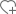关注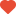已关注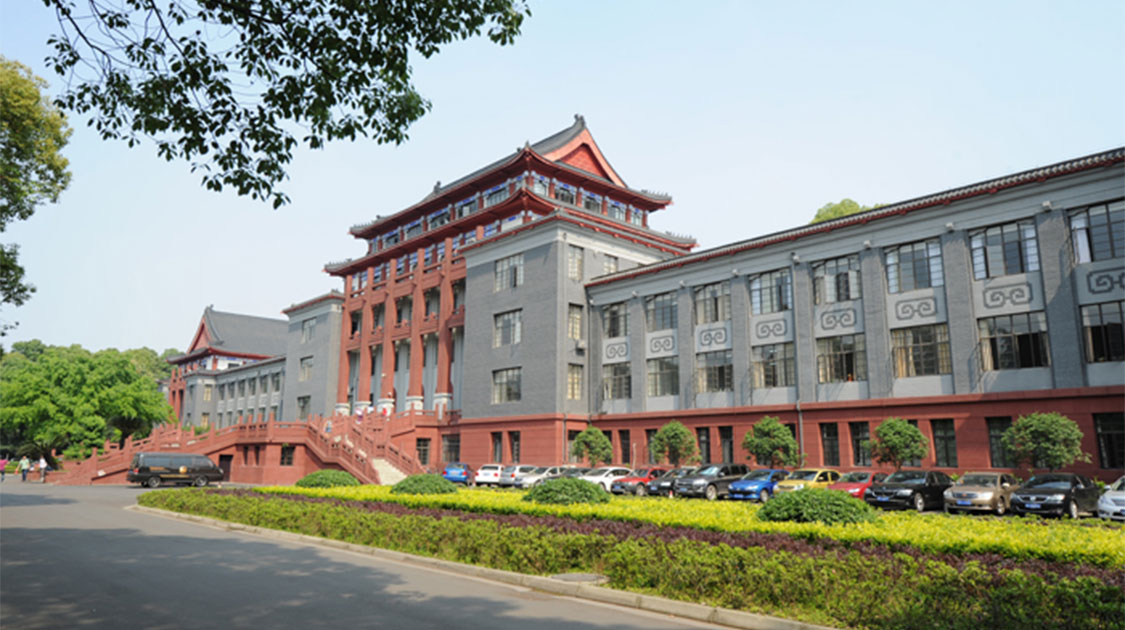•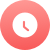1896

•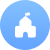公立

•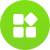综合

•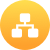教育部

•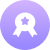本科

•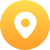四川-成都市

•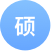443个

•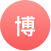399个

院校分数线

多方向招生特色专业

• 新闻学
• 动画
• 信息安全
• 核工程与核技术
• 水利水电工程
• 临床医学
• 口腔医学
• 汉语言文学
• 数学与应用数学
• 轻化工程
• 法医学
• 历史学
• 生物科学
• 高分子材料与工程
• 药学
• 机械设计制造及其自动化
• 水文与水资源工程
• 英语
• 化学
• 计算机科学与技术
• 护理学
• 制药工程
• 经济学
• 土木工程
• 基础医学
• 新能源材料与器件
• 医学信息工程院系/专业

• 经济学（本）
• 国民经济管理（本）
• 国际经济与贸易（本）
• 金融学（本）
• 金融工程（本）
• 财政学（本）
• 保险（本）

• 法学（本）

• 汉语言文学（本）
• 汉语国际教育（本）
• 新闻学（本）
• 广播电视学（本）
• 广告学（本）
• 编辑出版学（本）
• 网络与新媒体（本）

• 英语（本）
• 日语（本）
• 俄语（本）
• 法语（本）
• 西班牙语（本）

• 艺术设计（本）
• 动画（本）
• 绘画（本）
• 音乐学（本）
• 表演（本）
• 广播电视编导（本）
• 美术学（本）
• 舞蹈学（本）

• 历史学（本）
• 考古学（本）
• 文物与博物馆学（本）
• 会展经济与管理（本）
• 旅游管理（本）

• 数学与应用数学（本）
• 统计学（本）
• 信息与计算科学（本）

• 物理学（本）
• 应用物理学（本）
• 微电子学（本）
• 核工程与核技术（本）
• 核物理（本）

• 化学（本）
• 应用化学（本）
• 化学(基地班、试验班)（本）

• 生物科学（本）
• 生物科学(生物学基地)（本）
• 生物技术（本）
• 生物技术(生命科学与技术基地)（本）
• 生态学（本）
• 计算生物学(与软件学院联办)（本）

• 电子信息科学与技术（本）
• 电子信息工程（本）
• 电子科学与技术（本）
• 光信息科学与技术（本）
• 信息安全（本）

• 材料物理（本）
• 材料化学（本）
• 金属材料工程（本）
• 无机非金属材料工程（本）
• 生物医学工程（本）
• 新能源材料与器件（本）

• 机械设计制造及其自动化（本）
• 材料成型及控制工程（本）
• 工业设计（本）
• 测控技术与仪器（本）

• 电气工程及其自动化（本）
• 自动化（本）
• 通信工程（本）
• 医学信息工程（本）

• 软件工程（本）
• 计算机科学与技术（本）
• 网络工程（本）

• 建筑学（本）
• 城乡规划（本）
• 风景园林（本）
• 土木工程（本）
• 工程造价（本）
• 建筑环境与能源应用工程（本）
• 给排水科学与工程（本）
• 工程力学（本）
• 环境科学（本）
• 环境工程（本）

• 能源与动力工程（本）
• 水利水电工程（本）
• 水文与水资源工程（本）
• 农业水利工程（本）

• 化学工程与工艺（本）
• 过程装备与控制工程（本）
• 生物工程（本）
• 制药工程（本）
• 安全工程（本）
• 冶金工程（本）

• 纺织工程（本）
• 轻化工程（本）
• 服装与服饰设计（本）
• 食品科学与工程（本）
• 生物工程(轻工生物工程方向)（本）

• 高分子材料与工程(高分子材料方向)（本）
• 高分子材料与工程(加工工程方向)（本）

• 法医学（本）
• 基础医学（本）

• 临床医学(含5年制和8年制)（本）
• 护理学（本）
• 医学检验技术（本）
• 眼视光学（本）
• 医学影像技术（本）
• 康复治疗学（本）

• 口腔医学技术(四年制)（本）
• 口腔医学(五年制)（本）
• 临床医学(八年制)(口腔医学专业)（本）

• 预防医学（本）
• 卫生检验与检疫（本）
• 食品卫生与营养学（本）

• 药学(设药物化学、药学和临床药学三个专业方向)（本）

• 信息资源管理（本）
• 社会工作（本）
• 行政管理（本）
• 档案学（本）
• 信息管理与信息系统（本）
• 土地资源管理（本）
• 劳动与社会保障（本）
• 公共事业管理（本）
• 哲学（本）

• 管理科学（本）
• 工程管理（本）
• 工业工程（本）
• 工商管理（本）
• 人力资源管理（本）
• 市场营销（本）
• 电子商务（本）
• 会计学（本）
• 财务管理（本）
• 会计学(ACCA)（本）

• 航空航天工程（本）
• 飞行器控制与信息工程（本）

• 工业工程（本）
• 机械设计制造及其自动化（本）
• 材料科学与工程（本）

• 国际政治（本）

• 网络空间安全（本）

大学排名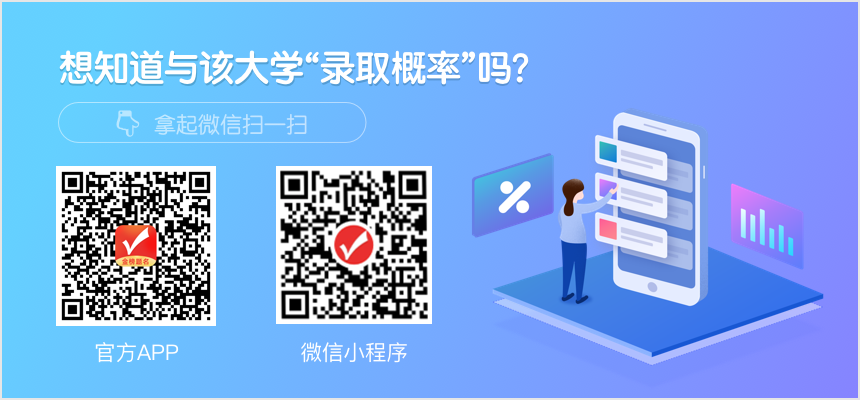知名校友

•李彦宏

•俞洪敏

•撒贝宁

•马云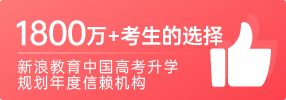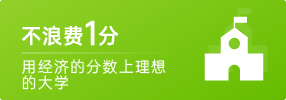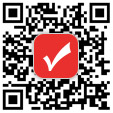400-181-5008

(免长话费 工作日 09:00-17:30)